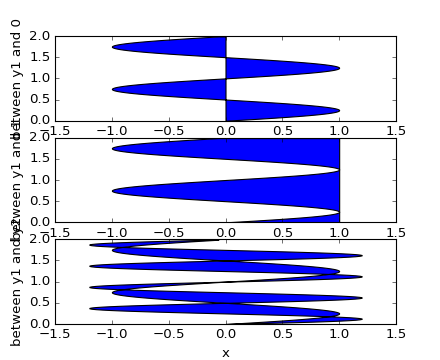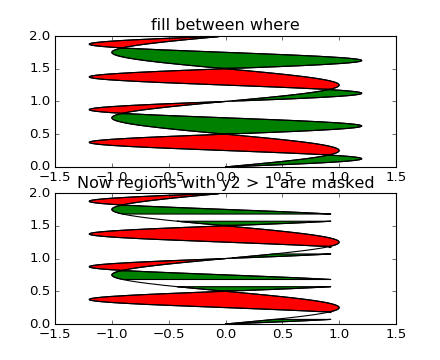### We're updating the default styles for Matplotlib 2.0

Learn what to expect in the new updatesTravis-CI:# pylab_examples example code: fill_betweenx_demo.py¶(png, hires.png, pdf)(png, hires.png, pdf)

```"""
Copy of fill_between.py but using fill_betweenx() instead.
"""
import matplotlib.mlab as mlab
from matplotlib.pyplot import figure, show
import numpy as np

x = np.arange(0.0, 2, 0.01)
y1 = np.sin(2*np.pi*x)
y2 = 1.2*np.sin(4*np.pi*x)

fig = figure()

ax1.fill_betweenx(x, 0, y1)
ax1.set_ylabel('between y1 and 0')

ax2.fill_betweenx(x, y1, 1)
ax2.set_ylabel('between y1 and 1')

ax3.fill_betweenx(x, y1, y2)
ax3.set_ylabel('between y1 and y2')
ax3.set_xlabel('x')

# now fill between y1 and y2 where a logical condition is met.  Note
# this is different than calling
#   fill_between(x[where], y1[where],y2[where]
# because of edge effects over multiple contiguous regions.
fig = figure()
ax.plot(y1, x, y2, x, color='black')
ax.fill_betweenx(x, y1, y2, where=y2 >= y1, facecolor='green')
ax.fill_betweenx(x, y1, y2, where=y2 <= y1, facecolor='red')
ax.set_title('fill between where')

# Test support for masked arrays.
ax1.plot(y1, x, y2, x, color='black')
ax1.fill_betweenx(x, y1, y2, where=y2 >= y1, facecolor='green')
ax1.fill_betweenx(x, y1, y2, where=y2 <= y1, facecolor='red')
ax1.set_title('Now regions with y2 > 1 are masked')

# This example illustrates a problem; because of the data
# gridding, there are undesired unfilled triangles at the crossover
# points.  A brute-force solution would be to interpolate all
# arrays to a very fine grid before plotting.

show()
```

Keywords: python, matplotlib, pylab, example, codex (see Search examples)